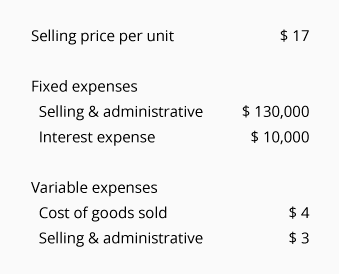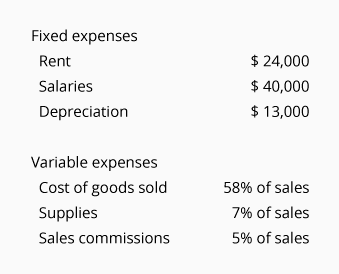# Break-even Point (Practice Quiz)

For multiple-choice and true/false questions, simply press or click on what you think is the correct answer. For fill-in-the-blank questions, press or click on the blank space provided.

1. 1.

Fixed Expenses do not change in total when there is a modest change in sales.

True
False
2. 2.

An example of a fixed expense would be a 5% sales commission.

True
False
3. 3.

Property taxes and rent are often fixed expenses.

True
False
4. 4.

Variable expenses change in total as volume changes.

True
False
5. 5.

An example of a variable expense is an office manager's monthly salary.

True
False
6. 6.

A retailer's cost of goods sold is an example of a variable expense.

True
False
7. 7.

Contribution margin is defined as sales (or revenues) minus variable expenses.

True
False
8. 8.

Break-even point is the point where revenues equal the total of all expenses including the cost of goods sold.

True
False
9. 9.

The break-even point in dollars of revenues is equal to the total of the fixed expenses divided by the contribution margin per unit.

True
False
10. 10.

If a company requires a profit of \$30,000 (instead of breaking even), the \$30,000 should be combined with the fixed expenses in order to compute the point at which the company will earn \$30,000.

True
False
11. 11.

If a company has mixed expenses, the fixed component can be combined with the company's fixed expenses and the variable component can be combined with the company's variable expenses when computing the break-even point.

True
False
12. 12.

Decreasing a company's fixed expenses should reduce the break-even point.

True
False
13. 13.

The contribution margin per unit is the selling price per unit minus the fixed expenses per unit.

True
False
14. 14.

Break-even analysis is useful for companies that sell products, but it is not useful for companies that provide services.

True
False
15. Use this information to answer questions 15 through 17:16. 15.

What is the company's contribution margin?

\$10
\$13
\$14
17. 16.

What is the break-even point in units?

10,000
14,000
20,000
18. 17.

If the company wants to earn a profit of \$42,000 instead of breaking even, what is the number of units the company must sell?

14,000
18,200
26,000
19. Use this information to answer questions 18 through 20:20. 18.

What is the company's contribution margin ratio?

30%
70%
Cannot Be Determined
21. 19.

What is the break-even point in dollars?

\$77,000
\$110,000
\$256,667
22. 20.

If the company wants to earn a profit of \$35,000 instead of breaking even, what is the amount of sales or revenue dollars the company must achieve?

\$112,000
\$145,000
\$373,333

Want more practice questions?